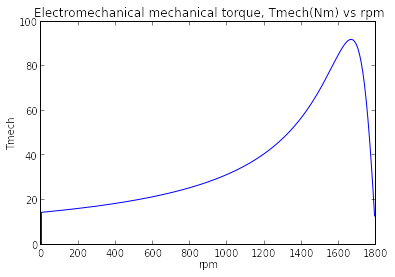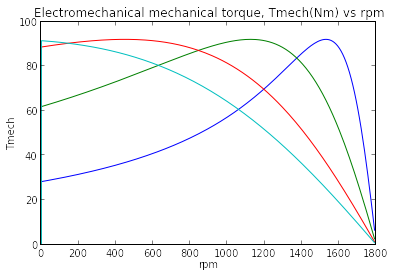# Chapter 6: Polyphase Induction Machines¶

### Example 6.1, Page number: 318¶

In :
from __future__ import division

#Variable declaration:
n=3502                              #Speed of motor(rpm)
Pin=15.7                            #Input power(kW)
Ia=22.6                             #Terminal current(A)
R=0.2                               #Stator winding resistance(ohm/ph)
f=60                                #frequency(Hz)
p=2                                 #No. of poles

#Calculations:
Ps=3*Ia**2*R/10**3                       #Power dissipated in stator winding(kW)
Pg=Pin-Ps                           #Air-gap power(kW)
ns=120*f/p
s=(ns-n)/ns
Pr=s*Pg                             #Power dissipated in stator(kW)

#Results:
print "Power dissipated in stator:",round(Pr*10**3,0),"W"

Power dissipated in stator: 419.0 W


### Example 6.2, Page number: 320¶

In :
from __future__ import division
import cmath
import math

#Variable declaration:
R1=0.294                            #Resistance of stator(ohm)
R2=0.144                            #Rotor resistance referred to stator(ohm)
X1=0.503                            #Reactance of stator(ohm)
X2=0.209                            #Reactance of rotor referred to stator(ohm)
Xm=13.25                            #Leakage reactance(ohm)
s=0.02                              #slip
Prot=403                            #Friction, windage and core losses(W)
V=220                               #Line-to-line voltage(V)
p=6                                 #No. of poles
fc=60                               #frequency(Hz)
nph=3                               #No. of phase

#Calculations:
Zf=((R2/s+1j*X2)*1j*Xm)/(R2/s+1j*X2+1j*Xm)
Zin=R1+1j*X1+Zf
V1=V/math.sqrt(3)
I1=V1/Zin
a=cmath.phase(I1)
pf=math.cos(a)
ns=120*fc/p
ws=4*math.pi*fc/p
n=(1-s)*ns
wm=(1-s)*ws
Pg=nph*abs(I1)**2*(Zf.real)
Psh=(1-s)*Pg-Prot
Tsh=Psh/wm
Pin=nph*(V1*I1).real
eff=Psh/Pin

#Results:
print "Rotor speed:     ",n,"rpm"
print "Output torque:   ",round(Tsh,2),"Nm"
print "Output power:    ",round(Psh,2),"W"
print "Stator current:  ",round(abs(I1),1),"A"
print "Power factor:    ",round(pf,3),"lagging"
print "Efficiency of motor:",round(eff*100,0),"%"

Rotor speed:      1176.0 rpm
Output torque:    42.4 Nm
Output power:     5221.6 W
Stator current:   18.8 A
Power factor:     0.846 lagging
Efficiency of motor: 86.0 %


### Example 6.3, Page number: 325¶

In :
from __future__ import division
import cmath
from math import *

#Variable declaration:
R1=0.294                            #Resistance of stator(ohm)
R2=0.144                            #Rotor resistance referred to stator(ohm)
X1=0.503                            #Reactance of stator(ohm)
X2=0.209                            #Reactance of rotor referred to stator(ohm)
Xm=13.25                            #Leakage reactance(ohm)
s=0.03                              #slip
V=220                               #Line-to-line voltage(V)
p=6                                 #No. of poles
fc=60                               #frequency(Hz)
nph=3                               #No. of phase

#Calculations:
#for part (a):
Zf=((R2/s+1j*X2)*1j*Xm)/(R2/s+1j*X2+1j*Xm)   #Impedance referred to stator(ohm)
Zin=R1+1j*X1+Zf                                 #Total input impedance(ohm)
Z1_eq=1j*Xm*(R1+1j*X1)/(R1+1j*(X1+Xm))          #Total equiv. impedance(ohm)
R1_eq=Z1_eq.real
X1_eq=Z1_eq.imag
V1=V/sqrt(3)
V1_eq=V1*(1j*Xm/(R1+1j*(X1+Xm)))
I2=abs(V1_eq)/sqrt((R1_eq+R2/s)**2+(X1_eq+X2)**2)
ws=4*pi*fc/p
ns=120*fc/p
Tmech=nph*I2**2*(R2/s)/ws
Pmech=nph*round(I2,1)**2*(R2/s)*(1-s)

#for part (b):
SmaxT=R2/sqrt(R1_eq**2+(X1_eq+X2)**2)     #slip at max torque
n_max=(1-SmaxT)*ns
Tmax=(1/ws)*(0.5*nph*abs(V1_eq)**2)/(R1_eq+sqrt(R1_eq**2+(X1_eq+X2)**2))

#for part (c):
s1=1                                        #Slip at starting of motor
I2_start=abs(V1_eq)/sqrt((R1_eq+R2)**2+(X1_eq+X2)**2)
Tstart=nph*I2_start**2*R2/ws

#Results:
print "(a) Load component I2 of stator current:",round(I2,1),"A"
print "    Electromechanical torque, Tmech    :",round(Tmech,1),"Nm"
print "    Electromechanical power, Pmech     :",round(Pmech,0),"W"

print "(b) Maximum electromechanical torque   :",round(Tmax,0),"Nm"
print "    Speed                              :",round(n_max,0),"rpm"

print "(c) Electromechanical starting torque Tstart:",round(Tstart,1),"Nm"
print "    Stator load current, I2_start           :",round(I2_start,0),"A"

(a) Load component I2 of stator current: 23.9 A
Electromechanical torque, Tmech    : 65.4 Nm
Electromechanical power, Pmech     : 7979.0 W
(b) Maximum electromechanical torque   : 175.0 Nm
Speed                              : 970.0 rpm
(c) Electromechanical starting torque Tstart: 77.6 Nm
Stator load current, I2_start           : 150.0 A


### Example 6.4, Page number: 328¶

In :
from __future__ import division
%matplotlib inline
import cmath
from math import *
from matplotlib import *
from pylab import *

#Variable declaration:
V=230                               #line to line voltage(V)
R1=0.095                            #Resistance of stator(ohm)
X1=0.680                            #Reactance of stator(ohm)
X2=0.672                            #Reactance of rotor referred to stator(ohm)
Xm=18.7                             #Leakage reactance(ohm)
f=60                                #frequency(Hz)
p=4                                 #No. of poles
nph=3                               #No. of phases

#Calculations and Results:
V1=V/sqrt(3)
omega=4*pi*f/p
ns=120*f/p
Z1eq=1j*Xm*(R1+1j*X1)/(R1+1j*(X1+Xm))           #Stator thevenin equivalent
R1eq=Z1eq.real
X1eq=Z1eq.imag
V1eq=abs(V1*1j*Xm/(R1+1j*(X1+Xm)))

print "Hence, the required plot is shown below:"
for m in range(1,6,1):                      #Loop over rotor resistance
if m==1:
R2=0.1
elif m==2:
R2=0.2
elif m==3:
R2=0.5
elif m==4:
R2=1.0
else:
R2=1.5

s=*202
rpm=*202
Tmech=*202
for n in range(1,201,1):                    #Loop over slip
s[n-1]=n/200                            #slip
rpm[n-1]=ns*(1-s[n-1])                  #rpm
I2=abs(V1eq/(Z1eq+1j*X2+R2/s[n-1]))     #I2
Tmech[n-1]=nph*I2**2*R2/(s[n-1]*omega)  #Electromechanical torque(Nm)

plot(rpm,Tmech)
title('Electromechanical mechanical torque, Tmech(Nm) vs rpm')
xlabel("rpm")
ylabel("Tmech")
if m==1:
show()
show()

Hence, the required plot is shown below:### Example 6.5, Page number: 335¶

In :
from __future__ import division
import math
from sympy import *
import math

#Variable declaration:
nph=3                       #No. of phases
k=0.429             #reactance ratio(X1/X2) from table 6.1,for class C motor
p=4                         #No.of poles
#Test 1: No-load test at 60 Hz
V1=219                      #Applied voltage, line-to-lne(V)
I1_nl=5.70                  #Phase current(A)
Pnl=380                     #Power(W)
ft=60                       #Hz

#Test 2: Blocked-rotor test at 15 Hz
V2=26.5                     #Applie voltage, line-to-line(V)
I1_bl=18.57                 #Phase current(A)
Pbl=675                     #Power(W)
fbl=15                      #Hz

#Test 3:
R1=0.262                    #Avg resistance per stator phase(ohm)

#Test 4: Blocked-rotor test at 60 Hz
V4=212                      #Applied voltage, line-line (V)
I2_bl=83.3                  #Avg phase current(A)
Pbl_4=20.1*10**3            #Power(W)
Tstart=74.2                 ##starting torque(Nm)

#Calculations:
#For part (a):
Prot=Pnl-nph*I1_nl**2*R1
V1_nl=V1/sqrt(3)                            #from test 1
Qnl=sqrt((nph*V1_nl*I1_nl)**2-Pnl**2)
Xnl=Qnl/(nph*I1_nl**2)
V1_bl=V2/sqrt(3)                            #from test 2
Qbl=sqrt((nph*V1_bl*I1_bl)**2-Pbl**2)
Xbl=(ft/fbl)*(Qbl/(nph*I1_bl**2))
X2=symbols('X2')
fx=k**2*X2**2+(Xbl*(1-k)-Xnl*(1+k))*X2+Xnl*Xbl
x=solve(fx,X2)
X2=round(x,2)                    #since X2 must be less than X1
X1=k*X2
Xm=Xnl-X1
Rbl=Pbl/(nph*I1_bl**2)
R2=(Rbl-R1)*((X2+Xm)/Xm)**2

#for part (b):
Pg=Pbl_4-nph*I2_bl**2*R1
ws=4*math.pi*ft/p
Tstart=Pg/ws

#Results:
print "\n  Equivalent ckt parameters:\n"
print"  R1=",round(R1,3),"ohm","  R2=",round(R2,3),"ohm"
print"  X1=",round(X1,3),"ohm","  X2=",round(X2,3),"ohm"," Xm=",round(Xm,2),"ohm"
print "\n(b) Starting torque:",round(Tstart,2),"Nm"

(a) N-load rotational loss: 354.0 W

Equivalent ckt parameters:

R1= 0.262 ohm   R2= 0.447 ohm
X1= 0.635 ohm   X2= 1.48 ohm  Xm= 21.2 ohm

(b) Starting torque: 77.7 Nm


### Example 6.6, Page number: 338¶

In :
from __future__ import division
from math import *

#Variable declaration:
Xnl=21.8                                #ohm
Xbl=2.01                                #ohm
R_1=0.26                                 #ohm
Rbl=0.65                                #ohm
V=220                                   #volt
#Here are the two sets of parameters
#Set 1 corresponds to the exact solution
#Set 2 corresponds to the approximate solution

R1=[0.262, 0.262]                        #ohm
R2=[0.447, 0.444]                        #ohm
X1=[0.633, 0.603]                        #H
X2=[1.47, 1.41]                          #H
Xm=[21.2, 21.2]                          #H
nph=3                                   #No. of phases
p=4                                     #No. of poles
Prot=354                                #Rotational losses(Watts)

#Calculations:
X_1=0.3*Xbl                              #(ohm) from table 6.1 and X1+X2=Xbl
X_2=Xbl-X_1                               #ohm
X_m=Xnl-X_1
R_2=(Rbl-R_1)*((X_2+X_m)/X_m)**2

#Results for part (a):
print "(a) The parameters:\n"
print"  R1=",round(R_1,3),"ohm","  R2=",round(R_2,3),"ohm"
print"  X1=",round(X_1,3),"ohm","   X2=",round(X_2,2),"ohm"
print"  Xm=",round(X_m,3),"ohm"

#Calculations & Results for part (b):
print "\n\n(b)"
#Here is the operating condition
V1=220/sqrt(3)
fe=60                                   #Hz
rpm=1746
#Calculate the synchronous speed:
ns=120*fe/p
ws=4*pi*fe/p
s=(ns-rpm)/ns
wm=ws*(1-s)
Zgap=*2
Zin=*2
Pmech=*2
I1=*2
I2=*2
Tmech=*2

#Calculate stator Thevenin equivalent:
#Loop over the two motors
for m in range(0,2,1):
Zgap = 1j*Xm[m]*(1j*X2[m] + R2[m]/s)/(R2[m]/s + 1j*(Xm[m] + X2[m]))
Zin=R1[m]+1j*X1[m]+Zgap
I1=V1/Zin
I2=I1*(1j*Xm[m])/(R2[m]/s+1j*(Xm[m]+X2[m]))
Tmech=nph*abs(I2)**2*R2[m]/(s*ws)   #Electromechanical torque
Pmech=wm*Tmech                      #Electromechanical power
Pshaft=Pmech - Prot
if (m==0):
print "Exact Solution:"
else:
print "\nApproximate Solution:"

print "\tPmech=",round(Pmech,1),"W","\tPshaft =",round(Pshaft,1), "W"
print "\tI1=", round(abs(I1),1),"A"

(a) The parameters:

R1= 0.26 ohm   R2= 0.443 ohm
X1= 0.603 ohm    X2= 1.41 ohm
Xm= 21.197 ohm

(b)
Exact Solution:
Pmech= 2820.7 W 	Pshaft = 2466.7 W
I1= 10.3 A

Approximate Solution:
Pmech= 2850.5 W 	Pshaft = 2496.5 W
I1= 10.4 A